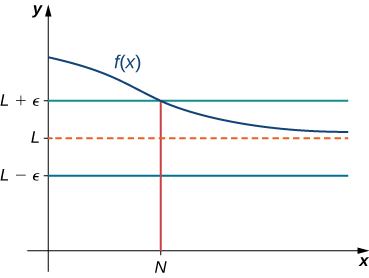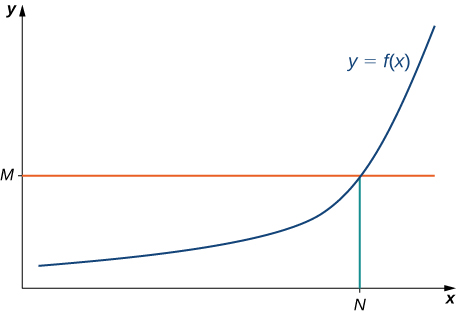# 4.6 Limits at infinity and asymptotes  (Page 3/14)

 Page 3 / 14

## Definition

(Informal) We say a function $f$ has an infinite limit at infinity and write

$\underset{x\to \infty }{\text{lim}}f\left(x\right)=\infty .$

if $f\left(x\right)$ becomes arbitrarily large for $x$ sufficiently large. We say a function has a negative infinite limit at infinity and write

$\underset{x\to \infty }{\text{lim}}f\left(x\right)=\text{−}\infty .$

if $f\left(x\right)<0$ and $|f\left(x\right)|$ becomes arbitrarily large for $x$ sufficiently large. Similarly, we can define infinite limits as $x\to \text{−}\infty .$

## Formal definitions

Earlier, we used the terms arbitrarily close , arbitrarily large , and sufficiently large to define limits at infinity informally. Although these terms provide accurate descriptions of limits at infinity, they are not precise mathematically. Here are more formal definitions of limits at infinity. We then look at how to use these definitions to prove results involving limits at infinity.

## Definition

(Formal) We say a function $f$ has a limit at infinity    , if there exists a real number $L$ such that for all $\epsilon >0,$ there exists $N>0$ such that

$|f\left(x\right)-L|<\epsilon$

for all $x>N.$ In that case, we write

$\underset{x\to \infty }{\text{lim}}f\left(x\right)=L$

We say a function $f$ has a limit at negative infinity if there exists a real number $L$ such that for all $\epsilon >0,$ there exists $N<0$ such that

$|f\left(x\right)-L|<\epsilon$

for all $x In that case, we write

$\underset{x\to \text{−}\infty }{\text{lim}}f\left(x\right)=L.$For a function with a limit at infinity, for all x > N , | f ( x ) − L | < ε .

Earlier in this section, we used graphical evidence in [link] and numerical evidence in [link] to conclude that $\underset{x\to \infty }{\text{lim}}\left(\frac{2+1}{x}\right)=2.$ Here we use the formal definition of limit at infinity to prove this result rigorously.

Use the formal definition of limit at infinity to prove that $\underset{x\to \infty }{\text{lim}}\left(\frac{2+1}{x}\right)=2.$

Let $\epsilon >0.$ Let $N=\frac{1}{\epsilon }.$ Therefore, for all $x>N,$ we have

$|2+\frac{1}{x}-2|=|\frac{1}{x}|=\frac{1}{x}<\frac{1}{N}=\epsilon$

Use the formal definition of limit at infinity to prove that $\underset{x\to \infty }{\text{lim}}\left(\frac{3-1}{{x}^{2}}\right)=3.$

Let $\epsilon >0.$ Let $N=\frac{1}{\sqrt{\epsilon }}.$ Therefore, for all $x>N,$ we have

$|3-\frac{1}{{x}^{2}}-3|=\frac{1}{{x}^{2}}<\frac{1}{{N}^{2}}=\epsilon$

Therefore, $\underset{x\to \infty }{\text{lim}}\left(3-1\text{/}{x}^{2}\right)=3.$

We now turn our attention to a more precise definition for an infinite limit at infinity.

## Definition

(Formal) We say a function $f$ has an infinite limit at infinity    and write

$\underset{x\to \infty }{\text{lim}}f\left(x\right)=\infty$

if for all $M>0,$ there exists an $N>0$ such that

$f\left(x\right)>M$

for all $x>N$ (see [link] ).

We say a function has a negative infinite limit at infinity and write

$\underset{x\to \infty }{\text{lim}}f\left(x\right)=\text{−}\infty$

if for all $M<0,$ there exists an $N>0$ such that

$f\left(x\right)

for all $x>N.$

Similarly we can define limits as $x\to \text{−}\infty .$For a function with an infinite limit at infinity, for all x > N , f ( x ) > M .

Earlier, we used graphical evidence ( [link] ) and numerical evidence ( [link] ) to conclude that $\underset{x\to \infty }{\text{lim}}{x}^{3}=\infty .$ Here we use the formal definition of infinite limit at infinity to prove that result.

Use the formal definition of infinite limit at infinity to prove that $\underset{x\to \infty }{\text{lim}}{x}^{3}=\infty .$

Let $M>0.$ Let $N=\sqrt{M}.$ Then, for all $x>N,$ we have

${x}^{3}>{N}^{3}={\left(\sqrt{M}\right)}^{3}=M.$

Therefore, $\underset{x\to \infty }{\text{lim}}{x}^{3}=\infty .$

Use the formal definition of infinite limit at infinity to prove that $\underset{x\to \infty }{\text{lim}}3{x}^{2}=\infty .$

Let $M>0.$ Let $N=\sqrt{\frac{M}{3}}.$ Then, for all $x>N,$ we have

$3{x}^{2}>3{N}^{2}=3{\left(\sqrt{\frac{M}{3}}\right)}^{2}{2}^{}=\frac{3M}{3}=M$

## End behavior

The behavior of a function as $x\to \text{±}\infty$ is called the function’s end behavior    . At each of the function’s ends, the function could exhibit one of the following types of behavior:

1. The function $f\left(x\right)$ approaches a horizontal asymptote $y=L.$
2. The function $f\left(x\right)\to \infty$ or $f\left(x\right)\to \text{−}\infty .$
3. The function does not approach a finite limit, nor does it approach $\infty$ or $\text{−}\infty .$ In this case, the function may have some oscillatory behavior.

A Function F(X)=Sinx+cosx is odd or even?
neither
David
Neither
Lovuyiso
f(x)=1/1+x^2 |=[-3,1]
apa itu?
fauzi
determine the area of the region enclosed by x²+y=1,2x-y+4=0
Hi
MP
Hi too
Vic
hello please anyone with calculus PDF should share
Which kind of pdf do you want bro?
Aftab
hi
Abdul
can I get calculus in pdf
Abdul
How to use it to slove fraction
Hello please can someone tell me the meaning of this group all about, yes I know is calculus group but yet nothing is showing up
Shodipo
You have downloaded the aplication Calculus Volume 1, tackling about lessons for (mostly) college freshmen, Calculus 1: Differential, and this group I think aims to let concerns and questions from students who want to clarify something about the subject. Well, this is what I guess so.
Jean
Im not in college but this will still help
nothing
how can we scatch a parabola graph
Ok
Endalkachew
how can I solve differentiation?
with the help of different formulas and Rules. we use formulas according to given condition or according to questions
CALCULUS
For example any questions...
CALCULUS
what is the procedures in solving number 1?
review of funtion role?
for the function f(x)={x^2-7x+104 x<=7 7x+55 x>7' does limx7 f(x) exist?
find dy÷dx (y^2+2 sec)^2=4(x+1)^2
Integral of e^x/(1+e^2x)tan^-1 (e^x)
why might we use the shell method instead of slicing
fg[[(45)]]²+45⅓x²=100
find the values of c such that the graph of f(x)=x^4+2x^3+cx^2+2x+2
anyone to explain some basic in calculus
I can
DebdootBy Jordon HumphreysBy Rylee MinllicBy OpenStaxBy OpenStaxBy OpenStaxBy John GabrieliBy Brooke DelaneyBy Jessica CollettBy Christine ZeelieBy Naveen Tomar